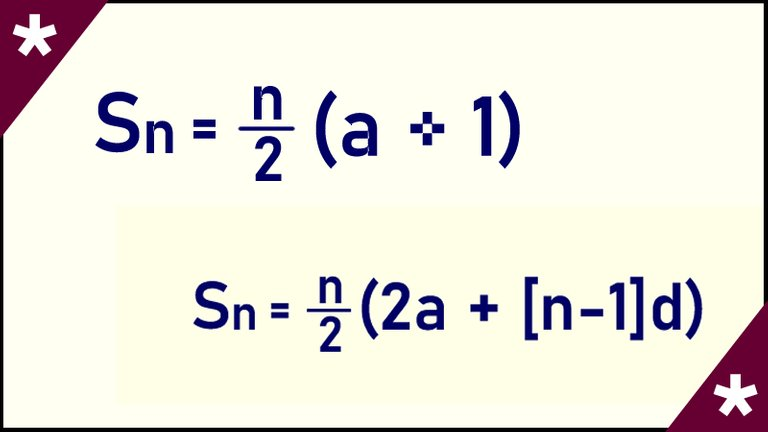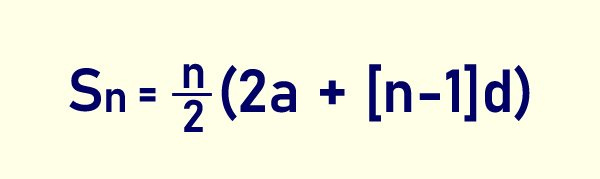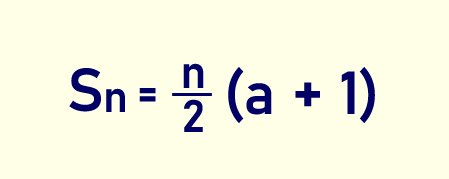# Finding the sum of terms of an Arithmetic Progression

in StemSocial4 months agoIts been a while I did my maths post. But am back. So last time out, I introduced Arithmetic Progression and I explained what it it. I was able to demonstrate how to solve for and find the nth term of an Arithmetic progression. We saw some examples. If you missed that post, you may want to take a look here.

This presentation will build on what we already know about Arithmetic progression. We want to find out how to find the Sum of Arithmetic progression.

## Formulas for finding the Sum of Arithmetic Progression

There are two standard formulas for finding the sum of arithmetic progression. Each formula is used depending on the make up of the arithmetic progression you are solving. So here are the two and when to use each:

### First FormulaIn this formula, here are the meaning of those alphabets used:
Sn = Sum of the terms of Arithmetic Progression
n = number of terms
a = The first term
d = The common difference

### Second FormulaThe main difference between the two formulas is that the first one contains d which is the common difference of the Arithmetic Progression. If the problem contains d, then formula one is used. Otherwise, the second formula is used.

Now we are going to demonstrate with two examples, on how to use the formulas to find the sum of Arithmetic Progression.

Example 1: Find the sum of 15 terms of the following Arithmetic Progression 6 + 8 + 10 +12 + 14

Solution
We will first check the items in the Arithmetic progression and know which formula yo use.
a = 6
d = 14 - 12 = 12 - 10 = 10 - 8 = 2
n = 15
l = 14

From the above, the first formula could be used because everything in it was supplied. So..

Sn = n/2(2a + [n-1]d)
Sn = 15/2(2 x 6 + [15-1]2) Now open the bracket inside
Sn = 7.5(12 + 14 x 2) Solving the figures inside
Sn = 7.5 (12 + 28)Expanding the main bracket
Sn = 7.5 x 40
Sn = 300

Example 2: Find the sum of 30th terms of the following Arithmetic Progression 13 + 11 + 9 + 7 + 5

Solution
First we have to determine the formula to use by looking at the problem again.

a = 13
d = 11 - 113 = 9 - 11 = 7 - 9 = -2
n = 30
l = 5

We can comfortably solve this with the first formula.
Sn = n/2(2a + [n-1]d)
Sn = 30/2(2 x 13 + [30-1]-2) Lets take care of the inside bracket
Sn = 15(26 + 29 x 2) Evaluating figures inside
Sn = 15(26 + 29 x 2) Still solving the bracket
Sn = 15(26 - 58) Expanding the bracket
Sn = 15 x (-32) Expanding the bracket
Sn = -480

## Conclusion

Notice that I have demonstrated using the first formula where the difference d is known. There are times when the d is unknown, then you can use the second formula. I will demonstrate that in the next post. But with the explanation and examples, one could easily find the sum or Arithmetic Progression easily especially when the common difference was given.

Sort:Thanks for your contribution to the STEMsocial community. Feel free to join us on discord to get to know the rest of us!

Please consider delegating to the @stemsocial account (85% of the curation rewards are returned).

Thanks for including @stemsocial as a beneficiary, which gives you stronger support.